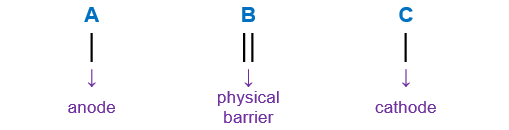# Problem: The line notation, Al(s) | Al3+(aq) || Co2+(aq) | Co(s), indicates thatA) Co is the reducing agent.B) Co2+ ions are oxidized.C) Al is oxidized.D) Al3+ is the reducing agent.E) Aluminum metal is the cathode.

###### FREE Expert Solution

We’re being asked to determine what the line notation, Al(s) | Al3+(aq) || Co2+(aq) | Co(s) indicates.

When writing a cell notation, we use the following format – “as easy as ABCRecall the mnemonics LEO GER.

95% (173 ratings)###### Problem Details

The line notation, Al(s) | Al3+(aq) || Co2+(aq) | Co(s), indicates that

A) Co is the reducing agent.

B) Co2+ ions are oxidized.

C) Al is oxidized.

D) Al3+ is the reducing agent.

E) Aluminum metal is the cathode.